# The Universe of Discourse

Mon, 14 Sep 2015

This is page 9 of the Cosmic Call message. An explanation follows.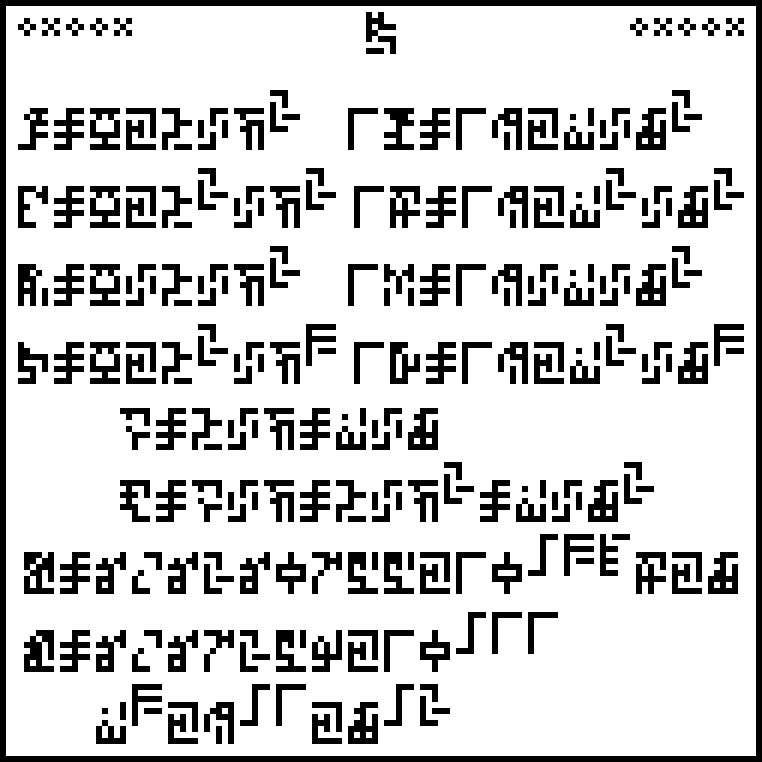The 10 digits are: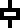0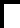1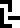2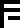3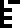4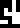5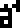6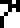7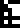8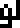9

The previous two pages defined fundamental units of mass, distance, and time. This page adds some derived notions: force, energy, pressure, and power; velocity and acceleration.

The first four lines are in two parts each. The left part defines an abstract quantity like force or energy; the right part defines a unit of that quantity like newtons or joules. For example, the second line defines energy and units of energy. The left side says that energy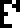is equal to mass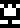times distance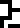squared divided by time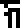squared. (This is the first appearance of the glyphs for distance and time.) The right side says that a joule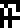of energy is !!1\frac{\mathrm{kg}\,\mathrm{m}^2}{\mathrm{s}^2}!!.

The two following lines define the abstract concepts of velocity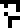(which has appeared before in connection with the speed of light) and acceleration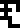(which is new). There are no units given for these.

 Concept Unit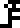forceenergy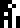pressure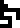powervelocityacceleration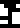newtonjoule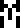pascal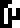watt (none) (none)

The newton and the joule won't appear again. Force won't appear again except on the last page, which asks “force WTF?”.

The final part of the page is the most interesting. It mentions the Planck constant !!h!!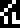(not the related !!\hbar!!) which is !!6.6260755\cdot 10^{{}^-34}!! joule-seconds and the universal gravitation constant !!G!!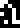which is !!6.67259\cdot 10^{{}^-11}\; \mathrm{m}^3\; \mathrm{kg}^{{}^-1}\; \mathrm{s}^{{}^-2}!!.

It's quite possible that the recipients won't recognize the gravitational constant, which is tricky to measure directly. Newton's law of universal gravitation was known on Earth for hundreds of years before the value of !!G!! was worked out. But if the recipients don't know it, they will be able to work it out from the later statements about the mass and radius of the Earth and the gravitational force at its surface, This would in turn allow them to calculate the mass of their own planet.

Note that on this page some inverse units are written with a division sign and some with a negative exponent. Will this inconsistency puzzle the aliens? My coworker Jeff Ober suggests that it communicates the important personal information that humans are confused and inconsistent.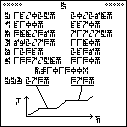The next article will discuss page 10, shown at right. (Click to enlarge.) Try to figure it out before then.